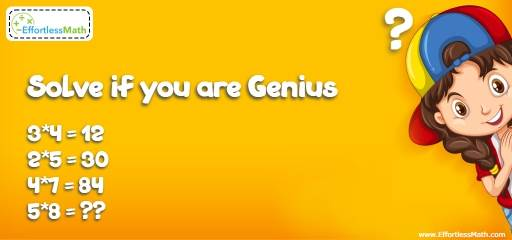# Algebra Puzzle – Critical Thinking 13

Can you solve this critical thinking challenge? This is a fun math puzzle for practicing children's math skills. It's also very useful for adults to challenge their brains!## Challenge:

3*4 = 12
2*5 = 30
4*7 = 84
5*8 = ??

### The Absolute Best Book to challenge your Smart Student!

Similar to critical thinking number 4, don’t try to find a relationship between numbers on both sides. Instead, consider the digits of each number and try to find a relationship between the two digits of the number in the left side. Try to find an operation between the digits of the number in the left side that can create the digits of the number of the ride side.
3 * 4 = 12
2 * 5 = 30
4 * 7 = 84
5 * 8 = ??
For the first equation, the product or 3 and 4 is 12, which is the answer.
For the second equation, the product of 2 and 5 is 10. But, the answer is 30. So, the symbol * is not a multiplication. 10 times 3 is 30.
Let’s find the pattern. We know that 3 * 4 = 12 and
2 * 5 = 30.
12 times what number is 12? It’s 1. So, 3 × 4 × 1 = 12 and    2 × 5 × 3 = 30
In the first equation, we should find number 1 from 3 and 4. In the second equation, we need to find 3 from 2 and 5. In fact, the difference of 3 and 4 is 1 and the difference of 2 and 5 is 3!
For the third equation, the product of 4 and 7 is 28. The difference of 4 and 7 is 3. Then:
4 × 7 × 3 = 84
Since the difference of 5 and 8 is 3, therefore, the answer to the last equation is:
5 * 8 = 5 × 8 × 3 = 120

### What people say about "Algebra Puzzle – Critical Thinking 13 - Effortless Math: We Help Students Learn to LOVE Mathematics"?

No one replied yet.

X
30% OFF

Limited time only!

Save Over 30%

SAVE $5 It was$16.99 now it is \$11.99